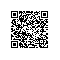# 剑指offer系列之七：斐波那契数列

package com.rhwayfun.offer;

public class Fibonacci {

public int getN(int n){
if(n == 0){
return 0;
}else if(n == 1){
return 1;
}

int one = 1;
int two = 0;
int sum = 0;
for (int i = 2; i <= n; i++) {
sum = one + two;
two = one;
one = sum;
}
return sum;
}

public static void main(String[] args) {
long a = new Fibonacci().getN(3);
System.out.println(a);
}
}使用钉钉扫一扫加入圈子
+ 订阅